# Low Pass Filter Transfer Function

Detailed mathematical analysis of passive low pass filter of the first and second order are presented.
The math involved in investigating the low pass filters of the first and second orders are very demanding. However a Low Pass Filter Transfer Function Graphing Calculator is included and may therefore be used for more practice.

  

## Impedances of Passive Components

The impedances $$Z_R$$ of a resistor with resistance $$R$$ is given by
$$\quad Z_R = R$$
The impedances $$Z_C$$ of a a capacitor with capacitance $$C$$ and the impedances $$Z_L$$ of a an inductor with inductance $$L$$ are given in complex form respectivley by:
$$\quad Z_C = \dfrac{1}{j \; \omega \; C} = \dfrac{1}{ s \; C}$$
$$\quad Z_L = j \; \omega \; L = s \; L$$
where $$s = j \; \omega$$

## Transfer Function of First Order Low Pass Filter

Let us consider the circuit below. As the frequency $$f$$ of the input signal $$v_{in}$$ decreases, the angular frequency $$\omega \; ( \; = \; 2\pi f )$$ decreases, the impedance of the capacitor $$Z_{C_1} = \dfrac{1}{ s \; C} = \dfrac{1}{ j \; \omega \; C}$$ increases and therefore the voltage across $$R$$ approaches zero and the voltage $$v_{out}$$ tends to a value close to $$v_{in}$$.
As the frequency increases, the impedance of the capacitor decreases to zero and therefore the output voltage $$v_{out}$$ tends to zero. Hence the RC circuit in Figure 1 allows the low frequencies only to pass through to the output. It is a low pass filter.

Let $$H = \dfrac{V_{out}}{V_{in}}$$ be the transfer function.
The circuit in Figure 1 is a voltage divider and using the impedances in complex form, we have

$$\qquad H(s) = \dfrac{Z_{C_1} }{Z_{C_1} + Z_{R_1}}$$

Substitute $$Z_{C_1} = \dfrac {1}{s \; C_1}$$ and $$Z_{R_1} = R_1$$ in the above to obtain

$$\qquad H(s) = \dfrac{ \dfrac {1}{s \; C_1} }{ \dfrac {1}{s \; C_1} + R_1}$$

and simplify to

$H(s) = \dfrac{ 1}{1 + R_1 \; C_1 \; s } \quad (I)$ or $H(\omega) = \dfrac{ 1}{1 + j \; R_1 \; C_1 \; \omega } \quad (II)$

Note that the order of a filter is given by the maximum power of $$s$$ in the transfer function. The maximum power of $$s$$ in (I) above is equal to $$1$$ and therefore the filter is of order 1.

$$H(\omega)$$ is a complex function in the form of the quotient of two functions

$$\qquad H(\omega) = \dfrac{Z_1}{Z_2}$$
According to complex numbers, the magnitude is given by
$\qquad |H(\omega)| = \dfrac{|Z_1|}{|Z_2|}$
and the argument (phase in ac circuits) is given by

Argumnent of $$H(\omega)$$ = Argument of $$Z_1$$ - Argument of $$Z_2$$

Note that the argument (in complex numbers) and phase (in ac circuit) are the same quantity.
The magnitude $$|H(\omega)|$$ and phase $$\Phi(\omega)$$ of the transfer function $$H(\omega)$$ in (II) above, are given by $|H(\omega)| = \dfrac{1}{\sqrt{1^2+(R_1 \; C_1 \; \omega)^2}} = \dfrac{1}{\sqrt{1+(R_1 \; C_1 \; \omega)^2}}$ $|\Phi(\omega)| = \arctan(0) - \arctan \left(\dfrac{R_1 \; C_1 \; \omega}{1}\right) = - \arctan \left(R_1 \; C_1 \; \omega \right)$

## The $$- 3$$ dB Cutoff Frequency of First Order Low Pass Filter

The cutoff angular frequency $$\omega_c$$ is defined as the frequency at which $|H(\omega_c)| = \dfrac{1}{\sqrt 2}$ Substitute $$|H(\omega_c)|$$ by its expression found above to obtain the equation $\dfrac{1}{\sqrt{1+(R_1 \; C_1 \; \omega_c)^2}} = \dfrac{1}{\sqrt 2}$ Square both side of the above equation and rewrite as $\dfrac{1}{1+(R_1 \; C_1 \; \omega_c)^2 } = \dfrac{1}{2}$ Solve to obtain the solution $\omega_c = \dfrac{1}{R_1 C_1}$

## Examples of First Order Low Pass Filter

Let $$R_1 = 100 \; \Omega$$ and $$C_1 = 1 \; \mu F$$
Substitute $$R_1$$ and $$C_1$$ by their numerical values to obtain
$\omega_c = \dfrac{1}{R_1 \; C_1} = \dfrac{1}{100 \times 1 \times 10^{-6} } = 10000 \; \text{ rad/s }$ $|H(\omega)| = \dfrac{1}{\sqrt{1+(10^{-4} \times \omega)^2}}$ $|\Phi(\omega)| = - \arctan \left( 10^{-4} \times \; \omega \right)$
Note that in order to better shows a flatness of the amplitude $$|H(\omega)|$$ over the frequencies allowed by the filter, we graph $20 \log_{10} (| H(\omega) |)$ whose unit is the decibel written as $$dB$$ on a semi-log graph.
At the cutoff frequency $$\omega = \omega_c$$, we have $|H(\omega_c)| = 20 \log_{10} \dfrac{1}{\sqrt{1+1}} = -3.01029 dB$ and $|\Phi(\omega_c)| = - \arctan ( 1) = - 45^{\circ}$
Note that $$\omega_c$$ is called the $$- 3 \text{ dB}$$ cutoff frequaency.

## Slope of the Graph for Large values of $$\omega$$

For large values of $$\omega$$, the term $$(R_1 \; C_1 \; \omega)^2$$ is much larger than $$1$$ and we therefore may make the following approximation. $| H(\omega) | = \dfrac{1}{\sqrt{1+(R_1 \; C_1 \; \omega)^2}} \approx \dfrac{1}{\sqrt{(R_1 \; C_1 \; \omega)^2}} \approx \dfrac{1}{R_1 \; C_1 \; \omega}$ For $$\omega = \omega_1$$, $| H_1(\omega) | \approx \dfrac{1}{R_1 \; C_1 \; \omega_1}$
For $$\omega = 10 \; \omega_1$$, $| H_2(\omega) | \approx \dfrac{1}{10 \; R_1 \; C_1 \; \omega_1}$
$20 \log_{10} | H_2 | - 20 \log_{10} | H_1 | = 20 \log_{10} \left( \dfrac{|H_2|}{|H_1|} \right) = 20 \log_{10} (\dfrac{1}{10}) = -20$
The factor $$10$$ is a decade and we, therefore, say the slope of the graph is $$-20 \; \text{dB}$$ per decade.
Points $$A$$ and $$B$$ on the graph below illustrate the above reasults. Point $$A$$ is at the frequency $$\omega_1 = 100000 \; \text{rad/s}$$ and Point $$B$$ is at the frequency $$\omega = 1000000 \; \text{rad/s} = 10 \; \omega_1$$. As we move from $$A$$ to $$B$$, the amplitude decreased by $$20 \; \text{dB}$$.
Note that this behavior happens for large values of $$\omega$$ above the cutoff frequency.

Below are shown the graph of $$20 \; \log_{10} H(\omega)$$ and the phase $$\Phi(\omega)$$.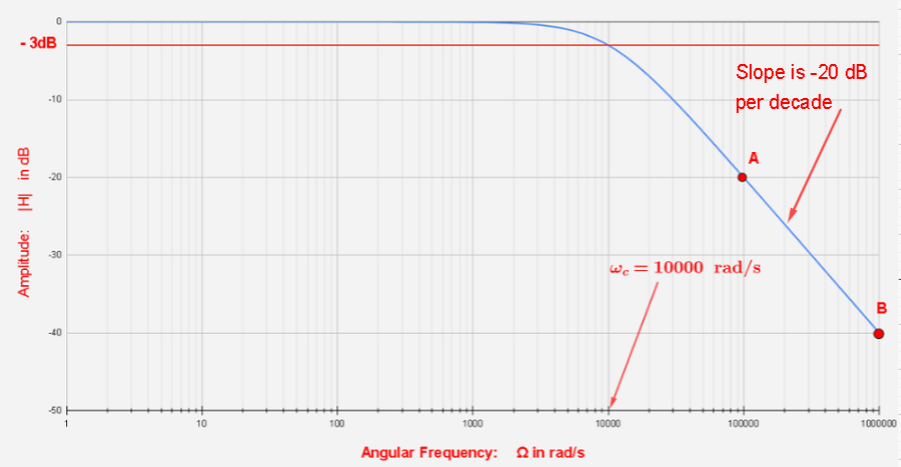Figure 2 - Transfer Function of First Order RC Low Pass Filter
The phase $$\Phi$$ is equal to $$-45^{\circ}$$ at $$\omega = \omega_c$$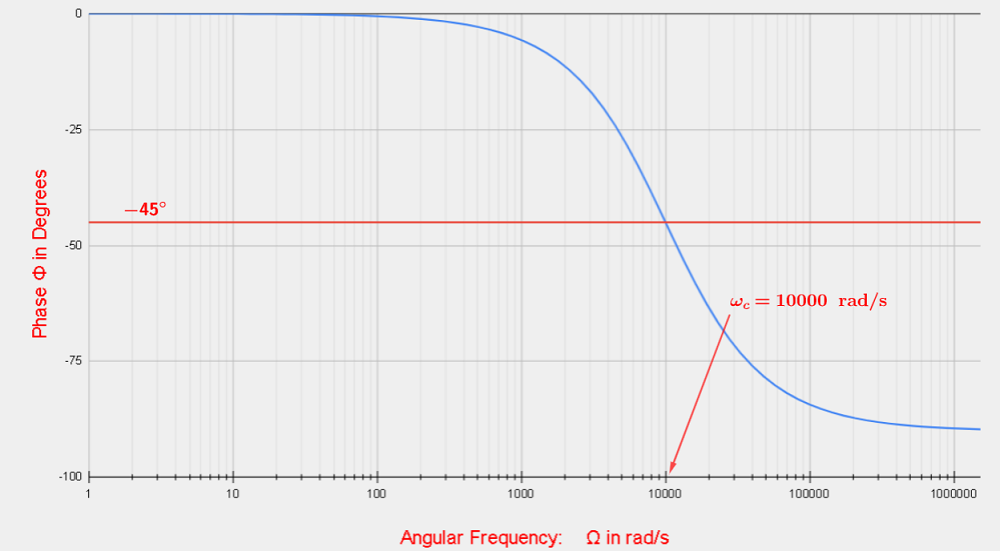Figure 3 - Phase of First Order RC Low Pass Filter

## Transfer Function of Second Order Low Pass Filter

We now analyse the transfer function of two cascaded low pass-filters.
In general, the transfer function $$H(s)$$ of the cascaded circuit shown below

is given by $H(s) = \dfrac{Z_4 \; Z_2 }{(Z_1 + Z_2)(Z_4 + Z_3 ) + Z_1 \; Z_2} \qquad (I)$
We now use the above formula (I) to find the transfer function of the second order low pass filter, which is a set of two cascaded low pass filters, as shown below.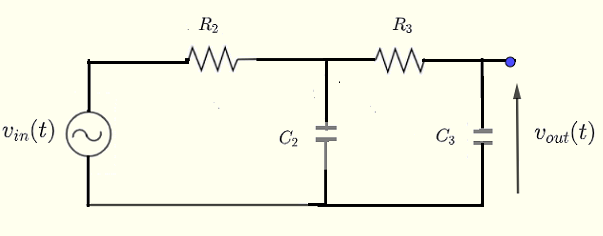Figure 5 - RC - RC Low Pass Filter of the Second Degree Substitute $$Z_2 = R_2$$, $$Z_3 = Z_{C_2} = \dfrac{1}{C_2 \;s}$$, $$Z_3 = R_3$$ and $$Z_4 = Z_{C_3} = \dfrac{1}{C_3 \;s}$$ in formula (I) above to obtain

$H(s) = \dfrac{1 }{ R_2 R_3 C_2 C_3 \; s^2 + (R_2 C_2 + R_3 C_3 + R_2 C_3) \; s + 1}$
Note that the highest power of $$s$$ is equal to $$2$$ and therefore the filter is of order $$2$$.

Substitute $$s = j \; \omega$$ and $$s^2 = - \omega^2$$ in $$H(s)$$ above to obtain
$H(\omega) = \dfrac{1 }{ 1 - R_2 \; R_3 \; C_2 \; C_3 \; \omega^2 + j \;(R_2 \; C_2 + R_3 \; C_3 + R_2 \; C_3 ) \; \omega }$
Let $A = R_2 \; R_3 \; C_2 \; C_3$ and $B = R_2 \; C_2 + R_3 \; C_3 + R_2 \; C_3$ and rewrite $$H(\omega)$$ as $H(\omega) = \dfrac{1 }{ 1 - A \omega^2 + j \;B \; \omega }$ The magnitude and phase of $$H(\omega)$$ are given by $| H(\omega) | = \dfrac{1}{\sqrt{ (1 - A\; \omega^2)^2 + (B\omega)^2 }}$
$\Phi (\omega) = - \arctan \left(\dfrac{ \;B \; \omega }{ 1 - A \omega^2 }\right)$

## The $$- 3 \text{ dB}$$ Cutoff Frequency of Second Order Low Pass Filter

The cutoff angular frequency $$\omega_c$$ is the frequency at which $|H(\omega_c)| = \dfrac{1}{\sqrt 2}$ Substitute $$|H(\omega_c)|$$ by its expression above to obtain the equation $$\dfrac{1}{\sqrt{ (1 - A\; \omega_c^2)^2 + (B\omega_c)^2 }} = \dfrac{1}{\sqrt 2}$$
Square both sides and rewrite the equation as
$$A^2 \omega_c^4 + (B^2 - 2 \; A) \; \omega_c^2 - 1 =0$$
The above equation has the quadratic form in $$\omega_c^2$$ and therefore has 4 solutions but only one is valid for this problem and is given by $\omega_c = \sqrt {\dfrac{{- B^2 + 2 \; A + \sqrt{B^4 - 4 B^2 \; A + 8 \; A^2}}}{2 \; A^2}}$
Rewrite the above solution as
$\omega_c = \dfrac{1}{\sqrt A} \sqrt {\dfrac{{-B^2 + 2 \; A + \sqrt{B^4 - 4 \; B^2 \; A + 8 \; A^2}}}{2 \; A}}$
which may be rewriten as
$\omega_c = \dfrac{1}{\sqrt A} \sqrt { - \dfrac{B^2}{2 \; A} + 1 + \sqrt{ \dfrac{B^4}{4 \; A^2} - \dfrac{4 B^2 \; A}{4 \; A^2} + 8 \dfrac{A^2}{4 A^2} } }$
Let $$r = \dfrac{B}{2 \sqrt A}$$ which gives $$B^2 = 4 \; A \; r^2$$ and $$B^4 = 16 \; A^2 \; r^4$$

Substitute $$B^2$$ and $$B^4$$ in the last expression of $$\omega_c$$ and simplify to obtain
$\omega_c = \dfrac{1}{\sqrt A} \sqrt { 1 - 2 r^2 + \sqrt{ 4 r^4 - 4 r^2+ 2 } }$

## Examples of Second Order Low Pass Filter

Let $$R_2 = 100 \; \Omega$$, $$C_2 = 1 \; \mu F$$, $$R_3 = 100 \; \Omega$$, $$C_3 = 1 \; \mu F$$

$$A = R_2 \; R_3 \; C_2 \; C_3 = 100 \times 1 \times 10^{-6} \times 100 \times 1 \times 10^{-6} = 1 \times 10^{-8}$$
and
$$B = R_2 \; C_2 + R_3 \; C_3 + R_2 \; C_3 = 100 \times 1 \times 10^{-6} + 100 \times 1 \times 10^{-6} + 100 \times 1 \times 10^{-6} = 3 \times 10^{-4}$$
$$r = \dfrac{B}{2 \sqrt A} = \dfrac{ 3 \times 10^{-4}}{2 \sqrt {1 \times 10^{-8}}} = 1.5$$
$\omega_c = \dfrac{1}{\sqrt { 1 \times 10^{-8}}} \sqrt { 1 - 2 (1.5)^2 + \sqrt{ 4(1.5)^4 - 4 (1.5)^2+ 2 } } = 3742.3 \; \text{rad/s}$

## Slope of the Graph for Large values of $$\omega$$

We first expand the expression in the denominator of $$| H(\omega) |$$ $| H(\omega) | = \dfrac{1}{\sqrt{ (1 - A\; \omega^2)^2 + (B\omega)^2 }} = \dfrac{1}{\sqrt{ 1 - 2 A \omega^2 + A^2 \omega^4 + B^2\omega^2 }}$ For large values of $$\omega$$, the term $$A^2 \omega^4$$ is much larger than all the terms under the square root in the denominator and we, therefore, may make the following approximation. $| H(\omega) | \approx \dfrac{1}{A \; \omega^2}$ For $$\omega = \omega_1$$, $| H_1(\omega) | \approx \dfrac{1}{A \; \omega_1^2}$
For $$\omega = 10 \omega_1$$, $| H_2(\omega) | \approx \dfrac{1}{A \; (10 \; \omega_1)^2}$
$$20 \log_{10} | H_2 | - 20 \log_{10} | H_1 | = 20 \log_{10} \left( \dfrac{|H_2|}{|H_1|} \right) = 20 \log_{10} (\dfrac{1}{100}) = -40$$
The factor $$10$$ is a decade and we, therefore, say the slope of the graph is $$-40 \; \text{dB}$$ per decade.

Below are shown the graph of $$20 \; \log_{10} H(\omega)$$ and the phase $$\Phi(\omega)$$.

Points $$A$$ and $$B$$ on the graph below illustrate the above reasults. Point $$A$$ is at the frequency $$\omega_1 = 100000 \; \text{rad/s}$$ and Point $$B$$ is at the frequency $$\omega = 1000000 \; \text{rad/s} = 10 \; \omega_1$$. As we move from $$A$$ to $$B$$, the amplitude decreased by $$40 \; \text{dB}$$.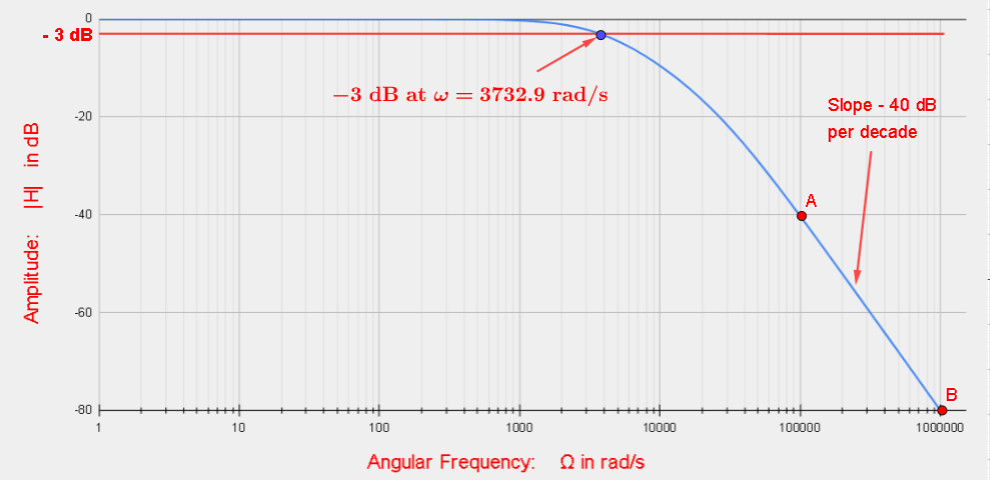Figure 2 - Transfer Function of Second Order RC Low Pass Filter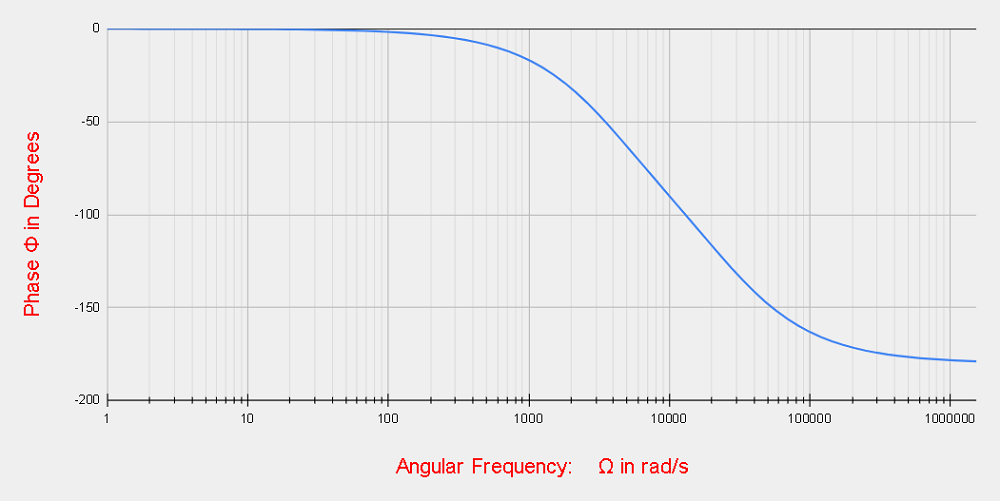Figure 3 - Phase of Second Order RC Low Pass Filter